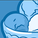# QlikView App Dev

Discussion Board for collaboration related to QlikView App Development.

Announcements
On May 18th at 10AM EDT we will answer your QlikView questions live. REGISTER
cancel
Showing results for
Did you mean:Contributor III

## Aggr. Unique Field in Load Script

Hi, I am trying to move the below expression to my LOAD SCRIPT, as the load times for this formula and the others like it (just using different week values) are simply too long. However, upon trying to re-create this in many different forms in the LOAD SCRIPT, I am still not getting the correct figures. I think the big issue I have, is that I am not Aggr., or Grouping by correctly in the LOAD SCRIPT. As can be seen from my expression, I want to Aggr. by "Product", but it was easier to do this here, because I had already loaded in all my other fields. In the LOAD SCRIPT, I need to not just include "Product", but also Customer & Type...but only Aggr. by "Product".

EXPRESSION TRYING TO RE-CREATE IN LOAD SCRIPT

=sum(aggr(

if(

sum(

aggr(sum(WK2_TOTAL),Product)

)

<

//TOTAL AMT OF WW1

Sum(

aggr(sum(WK1_TOTAL),Product)

)

,

//TOTAL AMT OF WW1

Sum(

aggr(sum(WK1_TOTAL),Product)

)

-

//TOTAL AMT OF WW9

Sum(

aggr(sum(WK2_TOTAL),Product))

,

0)

,Product)/1000000)

Here is what I am trying in the LOAD SCRIPT:

TABLE1:

Product,

Customer,

Type,

sum(WK1_TOTAL) as WK1_TOTAL,

sum(WK2_TOTAL) as WK2_TOTAL,

sum(WK3_TOTAL) as WK3_TOTAL

Resident

WK_TOTALS

Group by

Product,

Customer,

Type;

TABLE2:

Product,

Customer,

Type,

If(WK2_TOTAL < WK1_TOTAL,WK1_TOTAL - WK2_TOTAL,0) as CALCULATION1,

If(WK3_TOTAL < WK1_TOTAL,WK1_TOTAL - WK3_TOTAL,0) as CALCULATION2

Resident

TABLE1;

I have also tried

If(sum(WK2_TOTAL) < sum(WK1_TOTAL),sum(WK1_TOTAL) - sum(WK2_TOTAL),0) as CALCULATION1,

If(sum(WK3_TOTAL) < sum(WK1_TOTAL),sum(WK1_TOTAL) - sum(WK3_TOTAL),0) as CALCULATION2

Any help would be greatly appreciated! Thank you very much!

6 RepliesMaster II

Hi,

Can you attach some sample data for better understanding?MVP & Luminary

Maybe the expression itself could be optimized. You could try something like this:

sum(

aggr(if(

sum(WK2_TOTAL)<sum(WK1_TOTAL),

sum(WK1_TOTAL)-sum(WK2_TOTAL), 0),Product))

Beside this I'm not sure if you really need the aggr() - maybe something like: sum(TOTAL WK1_TOTAL) would be also working ...

- MarcusContributor III
Author

Hi Marcus,

Thanks for the response. However, I need to move the expressions to the load script, as I have the same expression being repeated many, many times...ultimately leading to unacceptable loading times. If I can compile the above IF statement into a value in the LOAD SCRIPT (essentially doing the If statement calculation itself in the script), I should be able to substitute my expression/formula, with just a single, calculated value...or as above CALCULATIONX.Contributor III
Author

Hi Arjun,

I'm sorry, I am unable to as the data in the script is confidential by my company...

However, I think the above data I posted should be enough in trying to determine a solution to write the IF statement in the LOAD SCRIPT?

Thanks!MVP & Luminary

I don't want to say that's not possible within the script but I rather doubt that you could get it calculated within a single field because your expression is a nested aggregation - at first against Product but then there will be quite probably further dimensions within your charts. Depending on the variety of views which you want to create you might need for each a special calculation.

Therefore I suggest to check if you really need the aggr-functions to get the desired results. For "normal" aggregations they aren't needed and beside adding complexity and more or less performance degrees they could also lead to wrong results. Please refer for this:

When should the Aggr() function NOT be used?

Pitfalls of the Aggr function

http://www.naturalsynergies.com/q-tip-14-aggr-and-synthetic-dimensions/

Should you could get your views without the aggr() your UI will be quite probably fast enough without a pre-calculation within the script.

- MarcusContributor III
Author

Thanks Marcus,

I'm still not certain of an answer just yet, but these links you sent helped me better understand my question a little better. I added a new discussion, with a refined question that hopefully contains my answer.

Thanks again.